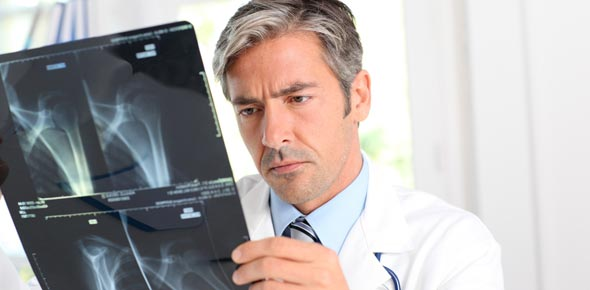# X-ray Tube And Control Panel For Urology Techs

32 Questions | Attempts: 861
ShareSettingsA quiz regarding the very basic X-ray parts needed to be known for Urology techs

• 1.
Primary function of the X-ray imaging system is to convert?
• A.

Electromagnetic energy to electric energy

• B.

Electric energy to electromagnetic energy

• C.

Electric energy to X-rays

• D.

Electromagnetic energy to X-rays

• 2.
Electric current is supplied where?
• A.

X-ray unit

• B.

Diode

• C.

Anode

• 3.
Where does the conversion take place?
• A.

X-ray tube

• B.

Glass envelope

• C.

Filament

• 4.
A Vacuumed tube or diode with two electrodes used to contain cathode and anode
• A.

Cathode

• B.

X-ray tube

• C.

Filament

• 5.
Where are the electron flow producing elements located?
• A.

Within the cathode

• B.

Within the anode

• C.

In the support structure

• 6.
What are the external structures of the X-ray tube?
• A.

Filament and Cathode

• B.

Glass envelope

• C.

Protective housing

• D.

Support structure

• E.

X-ray tube

• 7.
The Cathode The positive side of the X-ray tube and has two primary parts
• A.

True

• B.

False

• 8.
What is the Filament part of?
• A.

Cathode

• B.

Glass envelope

• C.

Diode

• D.

Anode

• 9.
Filament is what diameter?
• A.

2mm

• B.

2cm

• C.

2inches

• D.

2 meters

• 10.
How long is the filament?
• A.

1 to 2mm long

• B.

1 to 2cm long

• C.

1 to 2 inches long

• D.

4 to 6 inches long

• 11.
How many amps is needed to emit electrons from the filament?
• A.

At least 3

• B.

At least 4

• C.

At least 5

• D.

7 or more

• 12.
Surrounds the filament and helps contain the space charge
• A.

Anode

• B.

Disk weaver

• C.

Focal point

• D.

Focusing cup

• 13.
Directs the flow of electrons towards the anode
• A.

Focusing cup

• B.

Weaver's disk

• C.

Tungsten

• D.

Rotating Anode

• 14.
The Anode is positive
• A.

True

• B.

False

• 15.
Conducts electricity, radiates heat and contains the target
• A.

Anode

• B.

Filament

• C.

X-ray tube

• D.

Cathode

• 16.
What are the two types of anodes?
• A.

Stationary

• B.

Rotating

• C.

Mirror reverted

• D.

Divert

• 17.
Anodes must be?
• A.

Good thermal storage ability

• B.

Good thermal conductor

• C.

Mechanical support for target

• D.

Good electrical conductor

• E.

Has to have a melting point 3000 degrees C or higher

• 18.
Tungsten will melt at what degree?
• A.

3000 C

• B.

3000 F

• C.

3400 F

• D.

3400 C

• 19.
What is the anode made of?
• A.

Copper and brass

• B.

Tungsten and brass

• C.

Copper and Tungsten

• D.

Silver and Tungsten

• 20.
The target is were the electrons strike the anode
• A.

True

• B.

False

• 21.
Thermionic emission is electrons being boiled off and creates something called
• A.

Space emissions

• B.

Static charge

• C.

Space charge

• 22.
What is a advantage to a rotating anode?
• A.

Allows Electrons to interact with a larger surface area

• B.

Allows X-rays to interact with a much larger target area

• 23.
What is the focal point
• A.

The tip of the focal cup

• B.

The area on the target from which X-rays are emitted

• 24.
The smaller the focal spot, the better the spatial resolution of the image
• A.

True

• B.

False

• 25.
Allows the radiographer to control the X-ray tube current and voltage
• A.

Control booth

• B.

Control console

• C.

Operating console

• D.

Computer controls

## Related TopicsBack to top
×

Wait!
Here's an interesting quiz for you.